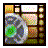# Joint Friction## Watch the video tutorial on using joint friction!Joint Friction Properties

Joint friction can be specified for the hinge and prismatic joint types. This allows you to create more realistic simulations of the movement around joints. If friction is not specified for a joint then any movement around that joint has the potential to keep going for a very long time. For example, if you create a simple pendulum with a hinge joint and apply a force to hit it then in the real world it will not take all that long for it to stop moving, but if no friction is applied then the pendulum will just keep right on swinging in the simulated environment.

Friction is determined by the Coefficient specified. By default friction is turned on for all new hinge and prismatic joints and they have a small coefficient of 0.02. You can turn friction on or off for a joint by using the Enabled property. The loss parameter allows you to specify the kinetic velocity loss for the joint. You can also specify the maximum friction force that can be applied to a joint. The proportional flag allows you to specify that the amount of friction force applied is proportional to the force on the joint. If this is false then the Coefficient parameter is no longer a coefficient, but is instead a constant force that is applied to retard the motion of the joint. Static and dynamic friction can be simulated using the static friction scale. This specifies the force ratio between static and dynamic friction.

## Joint Friction Properties

Enabled
Determines whether joint friction is applied or not.
Default value: True
Acceptable range:True or False.

Coefficient
The coefficient of friction to use for the joint.
Default value: 0.02
Acceptable range:Anything greater than or equal to 0.

Maximum Force
The maximum friction force that can be applied to the joint.
Default value: 10 N
Acceptable range:Anything greater than or equal to 0.

Loss
The kinetic loss for the joint
Default value: 0 s/Kg
Acceptable range:Anything greater than or equal to 0.

Proportional
If true then the friction force applied to the joint is proportional to the force on the joint. If false, then a constant friction force is applied.
Default value: True
Acceptable range:True or False.